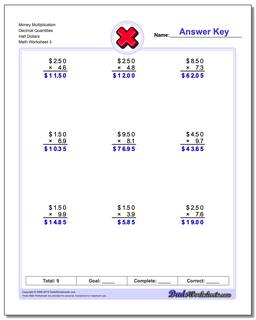One Dad. Four daughters. 9,193 worksheets... and counting!# Money Multiplication Decimal Quantities Half Dollars (Third Worksheet)

PropertyValue
DescriptionMoney Multiplication Decimal Quantities Half Dollars: Multiplication worksheets that multiply dollars times whole and decimal unit quantities. Some results will require rounding. (Third Worksheet)
Resource TypeWorksheet